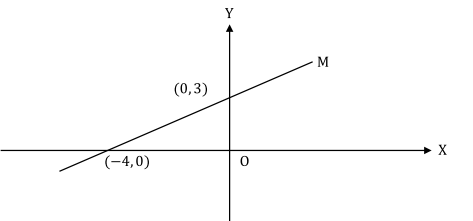# Free GRE Practice Questions

Question 1 of 1
ID: GRE-SMQ-9
Section: Quantitative Reasoning - Multiple Choice with one or more answer
Topics: Co-ordinate Geometry; Lines
Difficulty level: Medium

(Practice Mode: Single selected Question » Back to Overview)The graph of which of the following equations is a straight line that is parallel to line M in the figure above and intersects the negative direction of Y-axis?

Indicate all such equations.

[ Note: Select one or more answer choices ]

A$4y+3x=0$
B$4y-3x=-2$
C$4y-3x=4$
D$4y+3x=-4$
E$4y-3x=-1$
F$4y-3x=0$